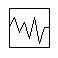# Controller -- Noise

Navigation: Models ➔ Control 2 Models ➔ Noise Controller

## General Description

• This unit operation is used to simulate process noise. It can be used to add disturbance to Feeders, pipe outlet capacity and other writeable variables.
• The Noise Control model is an orphan model, i.e it cannot be connected to any other unit operation.
• The noise model is for dynamic simulation only with the purpose of adding disturbance to the model.

### DiagramThe diagram shows the default drawing of the Noise control unit.

## Inputs and Outputs

There are no connections to this unit.

## Model Theory

In SysCAD 9.3 Build 136 or earlier, the random number generator used for these probability functions is from Numerical Recipes in C.

In SysCAD 9.3 Build 137 or later, the random number generator used for all probability functions is from the C++ standard library (http://www.cplusplus.com/reference/random/).

The Probability Density functions used for the Noise generation are shown in the following table. In all cases the following convention is used:

$\mu$ = Mean Value
$\sigma$ = Standard Deviation

For all methods, the tag PlantModel.RandomSeedAtStart (set on the Plant Model - FlwSolve page) affects the repeatability of the random values generated. For the same seed value, a repeatable set of random numbers will be generated. For a different seed, a different set of random numbers will be generated. If "*" is used, then a new random set of values will be generated on every run.

Type Probability Density Function P(t) NOTES
Gaussian
• $P(t) = \cfrac{1}{\sigma \sqrt{2\pi}}\cdot e^{\tfrac{-(t-\mu)^2}{2\sigma^2}}$
where $\sigma \gt 0$
Flat
• $P(t) = 0$
where $t \lt \mu - m$ or $t \gt \mu + m$
• $P(t) = \dfrac{1}{2\sigma}$

where $\mu - m ≤ t ≤ \mu + m$ and $m ≥ 0$ and $m$ = maximum deviation

Poisson
• $P(t) = \cfrac{\mu^t}{t!}\ e^{-\mu}$
where $e$ = the base of the natural logarithm system (2.71828...) and $\mu \gt 0$
Gamma
• $P(t) = \cfrac{1}{\Gamma(\alpha)\ \beta^{\alpha}}\ t^{\alpha-1}\ e^{-t/\beta}$

where $\alpha ≥ 0.5$ and $\beta \gt 0$

Note: The Chi-squared distribution is a special case of the Gamma distribution
with $\alpha = n/2$ and $\beta = 2$, where $n$ = degrees of freedom

Weibull
• $P(t) = \alpha^{-\beta}\ \beta\ t^{\beta-1}\ e^{-(t/\alpha)^\beta}$

where $t = \alpha (-\ln(U))^{1/\beta}$ and $\alpha ≥ 0.04$ and $\beta ≥ 0.01$
$U$ is a randomly generated number between 0 and 1

Bernoulli
• $P(t) = p^{t}\ (1-p)^{1-t}$

where $0 ≤ p ≤ 1$

(available in Build 137 or later)
Binomial
• $P(t) = \dbinom{n}{t} p^{t}\ (1-p)^{n-t}$

where $n$ is a positive integer, $\dbinom{n}{t} = \cfrac{n!}{t!(n-t)!}$ and $0 \lt p ≤ 1$

(available in Build 137 or later)
Geometric
• $P(t) = p\ (1-p)^{t}$

where $0 \lt p ≤ 1$

(available in Build 137 or later)
Exponential
• $P(t) = \mu\ e^{-\mu\ t}$

where $\mu \gt 0$

(available in Build 137 or later)
Extreme Value
• $P(t) = \cfrac{1}{\beta}\ z(t)\ e^{-z(t)}$
where $z(t) = e^{(\alpha-t)/\beta}$ and $\beta \gt 0$ (available in Build 137 or later)
Log Normal
• $P(t) = \cfrac{1}{s\ t\ \sqrt{2π}}\ e^{-(\ln t-m)^2/2s^2}$
where $0 \lt s ≤ 50.70$ (available in Build 137 or later)
Cauchy
• $P(t) = \cfrac{1}{π\ \beta\ \left [1+\left (\cfrac{t-\alpha}{\beta} \right )^2 \right ]}$
where $\beta \gt 0$ Example (available in Build 137 or later)
Fisher F
• $P(t) = \cfrac{Γ \left (\cfrac{m+n}{2} \right )}{Γ\left (\cfrac{m}{2}\right )\ Γ\left (\cfrac{n}{2}\right )}\ \frac{\left (\cfrac{m\ t}{n} \right )^{(m/2)}}{t\ \left (1+\cfrac{m\ t}{n} \right )^{(m+n)/2}}$
where $1 ≤ m ≤ 100$ and $n ≥ 1$ (available in Build 137 or later)
Student t
• $P(t) = \cfrac{1}{\sqrt{n\ π}}\ \cfrac{Γ\left (\cfrac{n+1}{2} \right )}{Γ\left (\cfrac{n}{2} \right )}\ \left (1+\cfrac{t^2}{n} \right )^{-(n+1)/2}$
where $n ≥ 0.25$ (available in Build 137 or later)